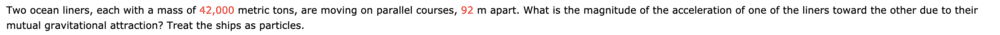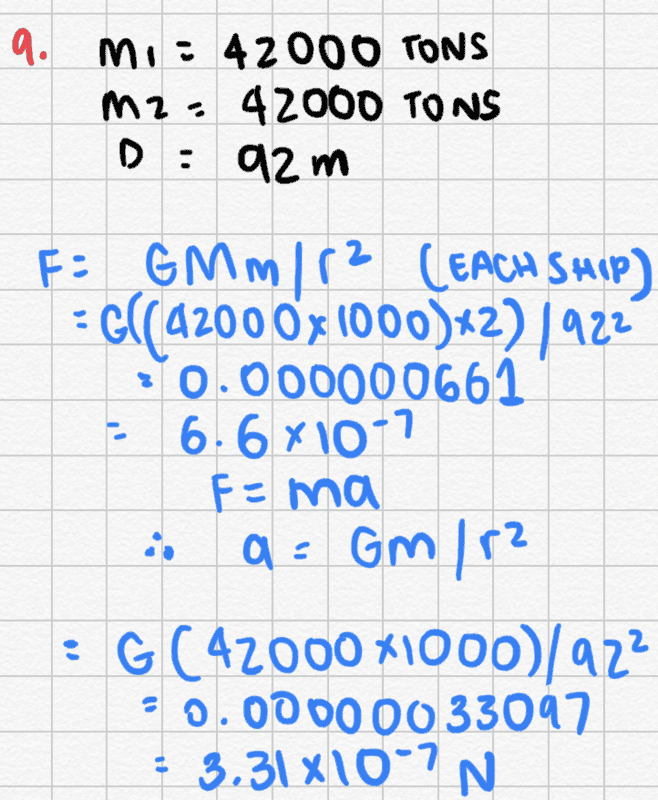# Calculating magnitude of the acceleration of two ocean liners

Homework Statement:
Two ocean liners, each with a mass of 42,000 metric tons, are moving on parallel courses, 92 m apart. What is the magnitude of the acceleration of one of the liners toward the other due to their mutual gravitational attraction? Treat the ships as particles.

I've calculated the Force but I'm struggling to calculate acceleration.
Relevant Equations:
F=GMm/r^2
F=maThis is what I've done so far:jbriggs444
Homework Helper
It is difficult to deal with large pictures of hand-drawn equations rather than with typewritten equations. Let us try to see what you did.

You started with $$\frac{GMm}{r^2}$$ and substituted in numbers for M, m and r yielding $$\frac{G (42000\times1000)^2}{92^2}$$

But I cannot figure out how you arrived at ##6.6\times10^{-7}## as a result.

Then you go back and apply ##F=ma## to the algebraic formula ##F=\frac{GMm}{r^2}## to correctly decide that $$a=\frac{Gm}{r^2}$$ You then evaluate this with m=42000000 and r=92 to obtain ##3.31\times10^{-7}##. But for some reason you decide that this result is a force rather than an acceleration and give it units of newtons.

mjc123
Homework Helper
But I cannot figure out how you arrived at 6.6×10−7 as a result.
He wrote and calculated (42000 x 1000) x2 instead of (42000 x 1000)^2

•jbriggs444
jbriggs444